Share

Books Shortlist
Your shortlist is empty

# RD Sharma solutions for Class 9 Mathematics chapter 9 - Triangle and its Angles

## Chapter 9 - Triangle and its Angles

#### Pages 9 - 10

Q 1 | Page 9

In a ΔABC, if ∠A = 55°, ∠B = 40°, find ∠C.

Q 2 | Page 9

If the angles of a triangle are in the ratio 1: 2 : 3, determine three angles.

Q 3 | Page 9

The angles of a triangle are (x − 40)°, (x − 20)° and (1/2x-10)^@. find the value of x

Q 4 | Page 9

The angles of a triangle are arranged in ascending order of magnitude. If the difference
between two consecutive angles is 10°, find the three angles.

Q 5 | Page 9

Two angles of a triangle are equal and the third angle is greater than each of those angles
by 30°. Determine all the angles of the triangle.

Q 6 | Page 9

If one angle of a triangle is equal to the sum of the other two, show that the triangle is a
right triangle.

Q 7 | Page 9

ABC is a triangle in which ∠A — 72°, the internal bisectors of angles B and C meet in O.
Find the magnitude of ∠BOC.

Q 8 | Page 9

The bisectors of base angles of a triangle cannot enclose a right angle in any case.

Q 9 | Page 9

If the bisectors of the base angles of a triangle enclose an angle of 135°, prove that the triangle is a right triangle.

Q 10 | Page 9

In a ΔABC, ∠ABC = ∠ACB and the bisectors of ∠ABC and ∠ACB intersect at O such that ∠BOC = 120°. Show that ∠A = ∠B = ∠C = 60°.

Q 11.1 | Page 9

Can a triangle have two right angles? Justify your answer in case.

Q 11.2 | Page 9

Can a triangle have two obtuse angles?  Justify your answer in  case.

Q 11.3 | Page 9

Can a triangle have two acute angles?Justify your answer in case.

Q 11.4 | Page 9

Can a triangle have  All angles more than 60°? Justify your answer in case.

Q 11.5 | Page 9

Can a triangle have All angles less than 60° Justify your answer in case.

Q 11.6 | Page 9

Can a triangle have All angles equal to 60°? Justify your answer in case.

Q 12 | Page 10

If each angle of a triangle is less than the sum of the other two, show that the triangle is acute angled.

#### Page 0

Two lines AB and CD intersect at O such that BC is equal and parallel to AD. Prove that the lines AB and CD bisect at O.

BD and CE are bisectors of ∠B and ∠C of an isosceles ΔABC with AB = AC. Prove that BD = CE.

#### Page 0

In two right triangles one side an acute angle of one are equal to the corresponding side and angle of the other. Prove that the triangles are congruent.

If the bisector of the exterior vertical angle of a triangle be parallel to the base. Show that the triangle is isosceles.

In a ΔABC, if ∠A=l20° and AB = AC. Find ∠B and ∠C.

In a ΔABC, if AB = AC and ∠B = 70°, find ∠A.

In Figure 10.24, AB = AC and ∠ACD =105°, find ∠BAC.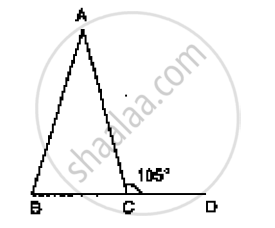Find the measure of each exterior angle of an equilateral triangle.

If the base of an isosceles triangle is produced on both sides, prove that the exterior angles so formed are equal to each other.

In Fig. 10.25, AB = AC and DB = DC, find the ratio ∠ABD : ∠ACD.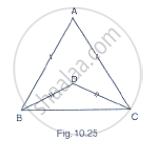Determine the measure of each of the equal angles of a right-angled isosceles triangle.

OR
ABC is a right-angled triangle in which ∠A = 90° and AB = AC. Find ∠B and ∠C.

AB is a line segment. P and Q are points on opposite sides of AB such that each of them is equidistant from the points A and B (See Fig. 10.26). Show that the line PQ is perpendicular bisector of AB.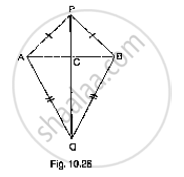#### Page 0

In Fig. 10.40, it is given that RT = TS, ∠1 = 2∠2 and ∠4 = 2∠3. Prove that ΔRBT ≅ ΔSAT.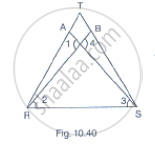In a ΔPQR, if PQ = QR and L, M and N are the mid-points of the sides PQ, QR and RP
respectively. Prove that LN = MN.

#### Page 0

Prove that the perimeter of a triangle is greater than the sum of its altitudes.

#### Page 0

In Fig. 10.131, prove that: (i) CD + DA + AB + BC > 2AC (ii) CD + DA + AB > BC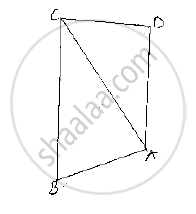## RD Sharma solutions for Class 9 Mathematics chapter 9 - Triangle and its Angles

RD Sharma solutions for Class 9 Maths chapter 9 (Triangle and its Angles) include all questions with solution and detail explanation. This will clear students doubts about any question and improve application skills while preparing for board exams. The detailed, step-by-step solutions will help you understand the concepts better and clear your confusions, if any. Shaalaa.com has the CBSE Mathematics for Class 9 by R D Sharma (2018-19 Session) solutions in a manner that help students grasp basic concepts better and faster.

Further, we at shaalaa.com are providing such solutions so that students can prepare for written exams. RD Sharma textbook solutions can be a core help for self-study and acts as a perfect self-help guidance for students.

Concepts covered in Class 9 Mathematics chapter 9 Triangle and its Angles are Inequalities in a Triangle, Some More Criteria for Congruence of Triangles, Properties of a Triangle, Criteria for Congruence of Triangles, Congruence of Triangles, Concept of Triangles.

Using RD Sharma Class 9 solutions Triangle and its Angles exercise by students are an easy way to prepare for the exams, as they involve solutions arranged chapter-wise also page wise. The questions involved in RD Sharma Solutions are important questions that can be asked in the final exam. Maximum students of CBSE Class 9 prefer RD Sharma Textbook Solutions to score more in exam.

Get the free view of chapter 9 Triangle and its Angles Class 9 extra questions for Maths and can use shaalaa.com to keep it handy for your exam preparation

S